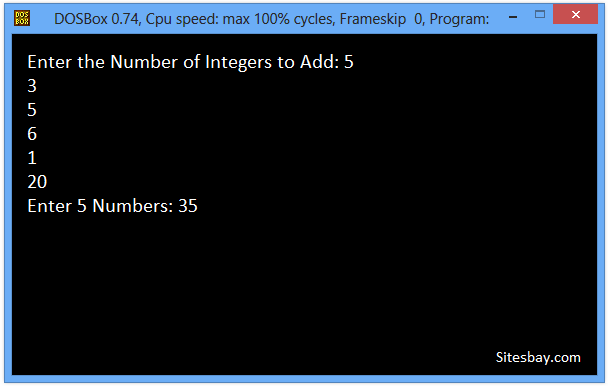# C++ Program to Find Sum of Natural Numbers

## Find Sum of Natural Numbers Program in C++

Natural Numbers are counting numbers, that is 1, 2, 3, 4, 5, 6, 7... etc. Remember, zero and negative numbers are not part of natural numbers. In this program, we will take a positive number N as input from user and find the sum of all natural numbers between 1 to N(including 1 and N).

## Example

```Enter the number of integers to add: 5
1 2 3 4 5
SUM = 15
```

To understand below example, you have must knowledge of following C++ programming topics; for looping statements we need know For Loop in C++ and Cout and Cin in C++.

## C++ program to find sum of numbers between 1 to N

```#include<iostream.h>
#include<conio.h>

void main()
{
int N=0, num, i, sum=0;
clrscr();
cout<<"Enter the Number of Integers to Add: ";
cin >> N;

cout<<"Enter "<<N<<" Numbers: ";
for(i= 0; i< N; i++){
cin>>num;
sum = sum + num;
}
cout<<"Sum is: "<<sum;

getch();
}
```

## Output

```Enter the Number of Integers to Add: 5
3
5
6
1
20
Enter 5 Numbers: 35
```## Pure VPN Privide Lowest Price VPN Just @ \$1.65. Per Month with Non Detected IP Lowest Price Non Detected IP VPN